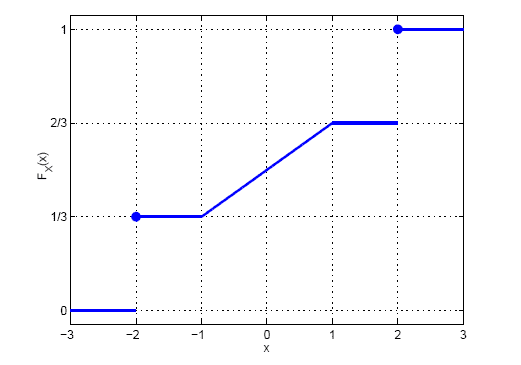# Conditional probability for random variable

• libelec

## Homework Statement

For the random variable X with the following cumulative distribution function:Calculate P(X$$\leq$$1.5|X<2), P(X$$\leq$$1.5|X$$\leq$$2) and P(X = -2| |X|=2)

## The Attempt at a Solution

This is an exercise about a subject I'm yet to see in class, but the teacher asked us to give it a try. I understand for conditional probability with random variables that P(X$$\leq$$a| X$$\leq$$b) = (P(X$$\leq$$a, X$$\leq$$b)/(P(X$$\leq$$b)), that is, the probability for the intesection of X$$\leq$$a and X$$\leq$$b divided by the probability of X$$\leq$$b.

For the first case, the intersection is X$$\leq$$1.5, and P(X$$\leq$$1.5) = 2/3 acording to the CDF. And P(X<2) is also 2/3, so P(X$$\leq$$1.5|X<2) = 1.

For the second case, the intersection is again X$$\leq$$1.5 and its probability is 2/3, but now, due to the jump in the function for X = 2, P(X$$\leq$$2) = 1. So P(X$$\leq$$1.5|X$$\leq$$2) = 2/3.

I don't know if what I'm doing is right or not. I've checked some text, but the exercises are mostly done through the distribution of probability function, not the CDF.

And for the third case I'm confused, since I have no idea how to calculate P(|X|= 2). Is it the sum of P(X=2) and P(X=-2) (since I can think |X|= 2 as (X=2 v X=-2), so the P(X=2 v X=-2) = P(X=2) + P(X=-2), taking into account that those are disjoint events)?

Thanks.

Anyone?

For the last part, you want the probability that X=2 given that |X|=2.

So there are two possibilities:
X=-2, X=2. These are disjoint events, each with a non-zero probability, and you want to know that given X is 2 or -2, what's the probability that it's two. There isn't really anything involving distributions here other than reading P(X=2) and P(X=-2) off of the graph

For the last part, you want the probability that X=2 given that |X|=2.

So there are two possibilities:
X=-2, X=2. These are disjoint events, each with a non-zero probability, and you want to know that given X is 2 or -2, what's the probability that it's two. There isn't really anything involving distributions here other than reading P(X=2) and P(X=-2) off of the graph

Right, so it's P(X=-2)/(P(X=-2) + P(X=2)) = (1/3)/(1/3 + 2/3) = 1/3. Is this OK?

And are the other calculations (and most importantly, the reasoning behind them) OK as well?

Thanks.

Except that the probability that X=2 is not 2/3